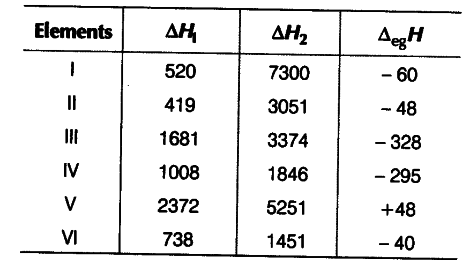# The first (∆iH1) and the second (∆iH2) ionisation enthalpies

The first (∆iH1) and the second (∆iH2) ionisation enthalpies (in kJ {{mol}^{-1}}) and the (∆egH) electron gain enthalpy (in kJ {{mol}^{-1}}) of a few elements are given belowWhich of the above elements is likely to be
(i) the least reactive element?
(ii) the most reactive metal?
(iii) the most reactive non-metal?
(iv) the least reactive non-metal?
(v) the metal which can form a stable binary halide of the formula { MX }_{ 2 }(X = halogen)?
(v) the metal which can form a predominantly stable covalent halide of the formula MX(X = halogen)?

(i) The least reactive element is element (V) because it has highest ∆iH1 (first ionisation enthalpy) and positive electron gain enthalpy (∆egH). The element (V) is an inert gas because inert gases have positive electron gain enthalpy. The given values for element (V) match with He.
{it) The most reactive metal is element (II) because it has the least ∆iH1 (first ionisation enthalpy) and low negative electron gain enthalpy (∆egH). The given values for element II match with K.
(iii) The most reactive non-metal is element (III) because it has high ∆iH1 (first ionisation enthalpy) and a very high negative electron gain enthalpy (∆egH). The given values for element III match with F.
(iv) The least reactive non-metal is element (IV). The element (IV) has not so high ∆iH1 but it has high negative electron gain enthalpy (∆egH). The given values for element (IV) match with (I).
(v) The metal which can form a stable binary halide of the formula MX2 is element (VI). Element (VI) is alkaline earth metal because it has low ∆iH1 but higher than that of alkali metals. Moreover, the difference between ∆iH1 and ∆iH2 very less. The given values for element (VI) match with Mg.
(vi) Element (I) has low ∆iH1 but a very high ∆iH2. It has less negative electron gain enthalpy. So, element (I) is alkali metal. The given values for element (I) match with Li. Lithium forms predominantly stable covalent halide of the formula MX.